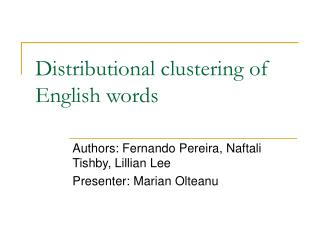DownloadDownload PresentationDistributional clustering of English words

# Distributional clustering of English words

Télécharger la présentation## Distributional clustering of English words

- - - - - - - - - - - - - - - - - - - - - - - - - - - E N D - - - - - - - - - - - - - - - - - - - - - - - - - - -
##### Presentation Transcript

1. Distributional clustering of English words Authors: Fernando Pereira, Naftali Tishby, Lillian Lee Presenter: Marian Olteanu

2. Introduction • Method for automatic clustering of words • Distribution in particular syntactic contexts • Deterministic annealing • Find lowest distortion sets of clusters • Increasing annealing parameters • Clusters subdivide – hierarchical “soft” clustering • Clusters • Class models • Word co-occurrence

3. Introduction • Simple tabulation of frequencies • Data sparseness • Hindle proposed smoothing based on clustering • Estimating likelihood of unseen events from the frequencies of “similar” events that have been seen • Example: estimating the likelihood of a particular direct object for a verb from the likelihood of that direct object for similar verbs

4. Introduction • Hindle’s proposal • Words are similar if there is strong statistical evidence that they tend to participate in the same events • This paper • Factor word association tendencies into associations of words to certain hidden classes and association between classes themselves • Derive classes directly from data

5. Introduction • Classes • Probabilistic concepts or clusters c • p(c|w) for each word w • Different than classical “hard” Boolean classes • Thus, this method is more robust • Is not strongly affected by errors in frequency counts • Problem in this paper • 2 word classes: V and N • Relation between a transitive main verb and the head noun of the direct object

6. Problem • Raw knowledge: • fvn– frequency of occurrence of a particular pair (v,n) in the training corpus • Unsmoothed probability - conditional density: • pn(v) = • This is p(v|n) • Problem • How to use pn to classify the nN

7. Methodology • Measure of similarity between distributions • Kullback-Leibler distance • This problem • Unsupervised learning – leardn underlying distribution of data • Objects have no internal structure, the only info. – statistics about joint appearance (kind of supervised learning)

8. Distributional Clustering • Goal – find clusters such that pn(v) is approximated by: • Solve by EM

9. Hierarchical clustering • Deterministic annealing • Sequence of phase transitions • Increasing the parameter β • Local influence of each noun on the definition of centroids

10. Results

11. Evaluation • Relative entropy • Where tn is the relative frequency distribution of verbs taking n as direct object in the test set

12. Evaluation • Check if the model can disambiguate between two verbs, v and v’